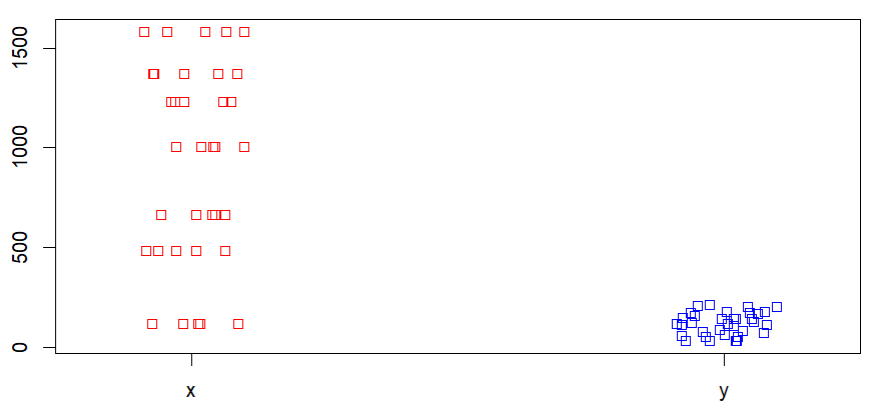Open In App

# How to Create a Strip Chart in R?

In this article, we will be throwing light on the various methodologies to create a strip chart using various functions and its arguments in the R programming language.

## Method 1:Create a Strip chart of the numeric vector

In this approach to create a strip chart of the numeric vector, the user needs to simply call the stripchart() function which is an inbuilt function of the R language. Then the user needs to pass the given vector for which the strip chart is needed and further by completing these previous steps the trip chart of the given vector will be returned.

stripchart() function: This function is used to create a strip chart of the given data.

Syntax: stripchart(vector)

Parameter: vector: It is the numeric vector

Example:

In this example, we are creating a strip chart of the vector containing 1000 random numeric values using the stripchart() function in the R programming language.

## R

 `# Create Data``x<-``c``(``rnorm``(1000))` `# Create strip chart``stripchart``(x)`

Output: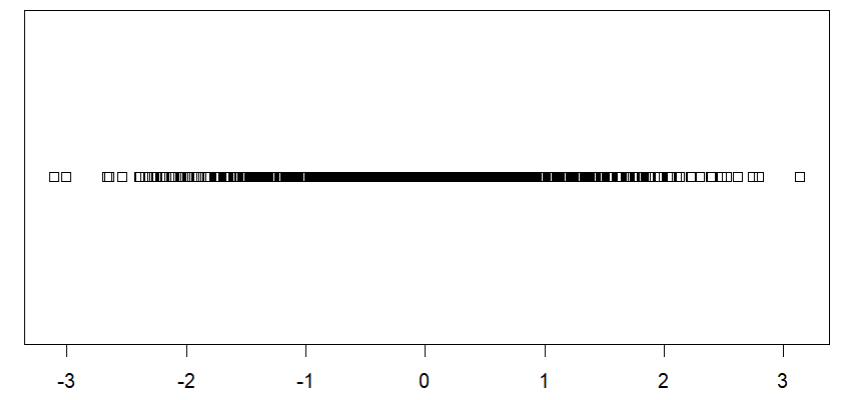## Method 2: Create a strip chart without overlapping of points

In this method of creating the strip chart without overlapping of points, the user needs to call the stripchart() function with the data to simply create the strip chart, and further, the user also needs to use the method argument of this function and set this as “jitter” to get the resulting strip chart with none overlapping individual points.

Syntax: stripchart(data,method=’jitter’)

Parameters:

• Data: Data provided to create the strip chart
• method: the method to be used to separate coincident points. The default method “overplot” causes such points to be overplotted, but it is also possible to specify “jitter” to jitter the points

Example: In this example, we are creating a strip chart without overlapping the points using the stripchart() function with the method argument set to be “jitter” in the R language.

## R

 `# Create Data``x<-``c``(``rnorm``(100))` `# Create strip chart``stripchart``(x,method=``'jitter'``)`

Output: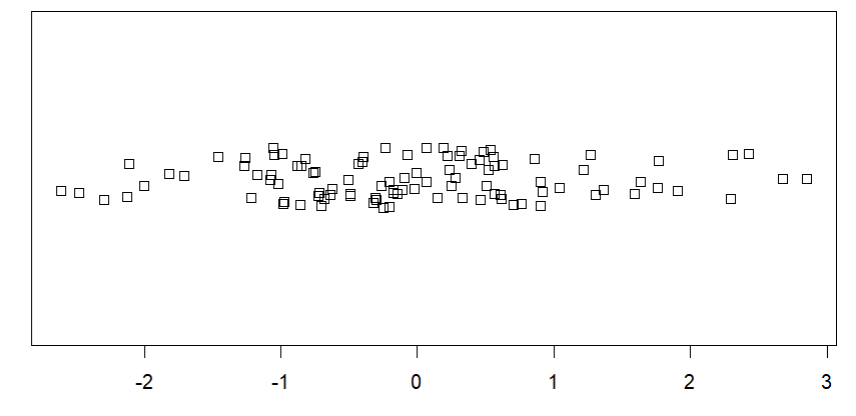### Create a strip chart without overlapping points with the method argument set to stack

We can also set the method parameter to be stacked in the case if the user needs to none overlapping individual points in the stripchart, here the points will be plotted as they are in stack in the R programming language

Syntax: stripchart(data,method=’stack’)

Parameters:

• Data: Data provided to create the strip chart
• method: the method to be used to separate coincident points.

Example: In this example, we are creating a strip chat without overlapping the points using the stripchart() function with the method argument set to be “stack” of the iris sepal width data in the R language.

## R

 `# Create Data``x<-Orange\$age` `# Create strip chart``stripchart``(x,method=``'stack'``)`

Output: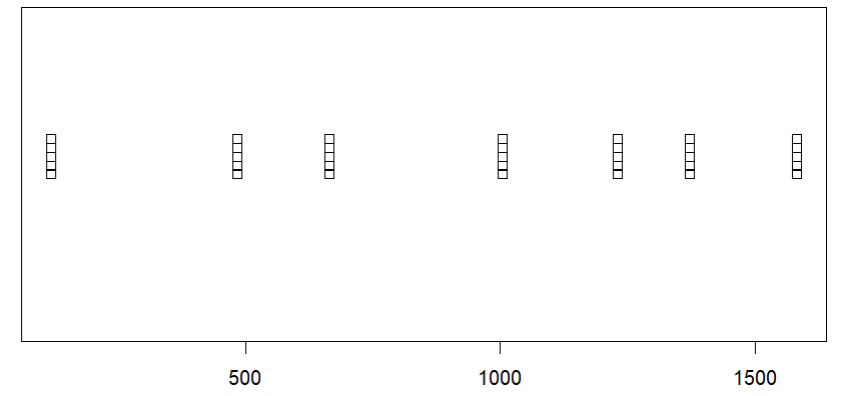## Method 3: Create a vertical strip chart

Under this method to create a vertical strip chart, the user needs to call the stripchart() function with its vertical argument, where the vertical argument should be initialized to true as by default if the value is false to get the resulting strip chart as a vertical strip chart of the given data in R language.

Syntax: stripchart(data,method,vertical=TRUE)

Parameters:

• Data: Data provided to create the strip chart
• method: the method to be used to separate coincident points.
• vertical: when vertical is TRUE the plots are drawn vertically rather than the default horizontal.

Example: In this example, we are creating a vertical strip chart using the vertical argument of the stripchart() function of the given data in the R programming language.

## R

 `# Create Data``x<-``c``(``rnorm``(100))` `# Create strip chart``stripchart``(x,method=``'jitter'``,vertical = ``TRUE``)`

Output: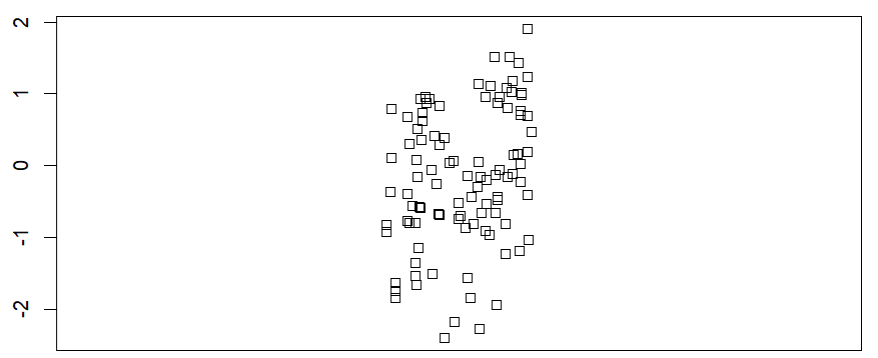## Method 4: Create strip chart for multiple data

In this approach to create the strip chart of the multiple data, the user simply has to create a list of the multiple data together in a single variable and then pass that variable as the parameter of the stripchart() function to get the strip chart of the all the provided data in R language.

Example: In this example, we are creating a strip chart of the multiple data provided in the method jitter and in the vertical form using the stripchart() function in the R language.

## R

 `# Create Data``gfg<-``list``(x=Orange\$age,y=Orange\$circumference)` `# Create strip chart``stripchart``(gfg,method=``'jitter'``,``           ``col=``c``(``'red'``,``'blue'``),``           ``vertical = ``TRUE``)`

Output: